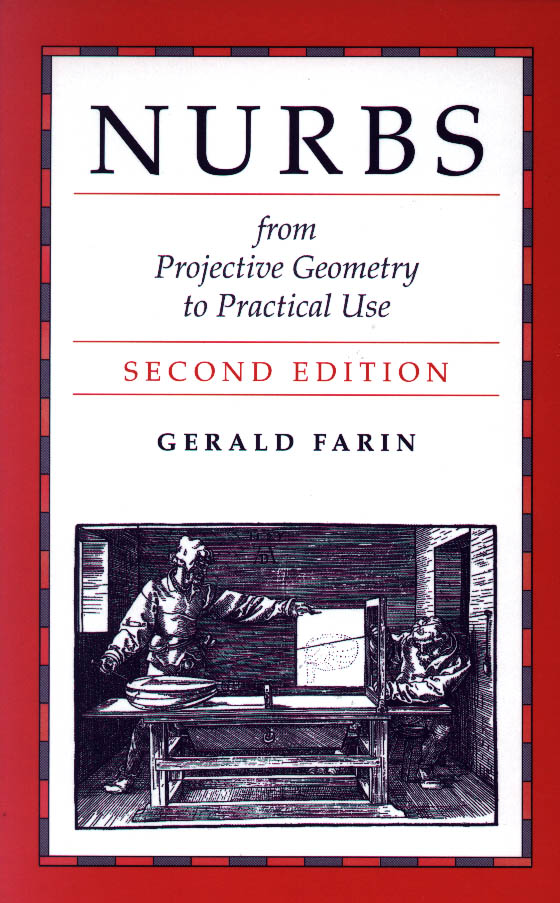# NURBS for Curve and Surface Design from Projective Geometry to Practical Use 2nd edition

by Gerald Farin

Published in 1999 by AK Peters, Ltd.
267 Pages
ISBN 1-56881-084-9

Errata

Please send email with suggestions/corrections to farin@asu.edu. If you find an error, you'll get credit in this Errata page!
In 2013, a new printing will be released and most of these errata should be fixed in this version. Check the printing date of your book.

• p. XIV, middle: Bezier curves curves should be Bezier curves. (M. Ferrara)

• p.2, footnotes 2-5 were meant to be index entries, not footnotes.

• p. 12, "any four points a,b,c,s" should be "any four points a,b,c,d." (M. Phielipp)

• p. 13 eq (1.13) should read x =[ [[a+ug]\wedge f]\wedge [[a+vf]\wedge g] ] (Justin Leonard)

• p. 47, Fig. 3.5: the line Y should go through a marked point ${hat A} \wedge X$ (M. Phielipp)

• p. 60, paragraph after (4.1): the term [x,x',x'] should really be [x,x',x''], with ' meaning "dot". (S. Lanham and A. Gustafsson)

• p. 66, "first derivative curve" should be "first derivative line". Same for caption to Fig. 4.6.

• Page 94: See (2.8) for a definition of cross RATIOS. (M. Ferrara)

• Page 94: second displayed equation: and m_1 (M. Ferrara)

• Page 95: Equation (6.1) +\frac{\Delta_{0}}{\Delta_{0}+\Delta_{1}}b_{3} (M. Ferrara)

• p. 108, second displayed equation: "ldots" should be "..."

• p. 110, middle: it is "pushed away".. (M. Ferrara)

• Page 112: Third paragraph:  being positive, the... (M. Ferrara)

• page 113, (7.7)subscript +1 should be i+1 (C.de Boor)

• page 123, The term "improperly parametrized" in the middle of the the page is not used correctly. It should refer to "degenerate curves" instead. An example of an improperly parametrized curve is given by the Bezier curve on page 172. A curve is improperly parametrized if each point on the curve corresponds to several parameter values. A better reference than  is: T. Sederberg: Degenerate parametric curves. CAGD 1 (4): 301-307, 1984. (J. Sanchez-Reyes)

• page 127, (7.39): lots of braces missing around those intended superscripts. (C. de Boor)

• page 134, bottom: The claimed convergence of the rational part to a point will not always take place. (J. Sanchez-Reyes). For a proof, see Wang, G.J., Sederberg, T.W., Chen, F. (1997). On the Convergence of Polynomial Approximation of Rational
Functions. Journal of Approximation Theory 89, 267-288.

• Page 148, first sentence: zeroes of the denominator. (M. Ferrara)

• Page 150: Section 8.7:  given curvatures at b_{0} and b_{3}. (M. Ferrara)

• Page 155, (9.1): u_r should be u_{I+1}. (M. Ferrara)

• Page 158: Sentence 1: The curve segment from Fig (M. Ferrara)

• page 160: Figure 9.7 is wrong. The correct one is in the ps-directory.

• Page 160: Sentence 1: ... and [u_{i}, u_{i+1}]  (M. Ferrara)

• page 164, first displayed ewuation: needs to be a superscript(M. Ferrrara)

• page 164, sentence after displayed equation: delete. (M. Ferrrara)

• Page 165: Section 9.7  lies in the convex hull of  (M. Ferrrara)

• page 164, footnote: "B-splines" should be "B-spline curves" (C. de Boor)

• Page 177: Equation (10.14) Extra parenthesis on the right hand side. (M. Ferrara)

• Page 178: Section 10.5: The 3D caseon the unit sphere. (M. Ferrara)

• 183: Footnote: See section 11.5. (M. Ferrara)

• Page 185: Second bullet: n and m, respectively. (M. Ferrara)

• Page 186: Caption: w_{1} (M. Ferrara)

• Page 195: Equation (11.18): Delete the extra =. (M. Ferrara)

• Page 198: Fig. 11.14 gives. (M. Ferrara)

• Page 203: Sentence 2: {a_{i}} and {c_{i}} (M. Ferrara)

• Page 204: Sentence 2: a_{2} (M. Ferrara)

• Page 204: Paragraph 6, Sentence 2:  conic surface, (M. Ferrara)

• Page 210: Figure 12.3 caption: If *we* increase (M. Ferrara)

• Page 212: First sentence of last paragraph:  midpoints (M. Ferrara)

• Page 224: Second paragraph: First sentence: \underline{b}_{101} (M. Ferrara)

• page 225, first displayed equation: the b_i should be underlined. (M. Ferrrara)

• page 225, eq. (13.4): \underline{x} should be \underline{u} (M. Ferrrara)

• page 227, example 13.1: first sentence: Its coefficients. Delete the "w" in the example. (M. Ferrrara)

• Page 234: Figure 14.1: The pair of points is not marked by squares as referenced in the text. These are the points which are labeled in Fig. 14.2. (M. Ferrrara)

• Page 238: Section 14.4: Bezier based (M. Ferrrara)

• page 243: u_{L+n} should be u_{N+n} (C. de Boor)

• page 245, second bullet: strictly speaking, the curve with the second sequence of knots would be nonzero for t in (-10,10), and would start and finish at the origin, while the first would be nonzero only for t in [0,1] and would not, in general start and finish at the origin. (C. de Boor)

• p. 249: The second entry in the first column of the fourth row of columns should be d and not -d ; there is also an extra ( in the last entry of the middle column in both row 2 and 4. (C. de Boor and R. Tamstorf)

• p. 251: delete second definition of "wedge". (M. Ferrara)

• Page 253:  : 15(7):675-697. (M. Ferrara)

• Page 256 : IBM Journal.... (M. Ferrara)

• Page 258:   quadric precision for (M. Ferrara)

• Page 259:  rational Bezier form (M. Ferrara)

• Page 262: A recursive algorithm (M. Ferrara)